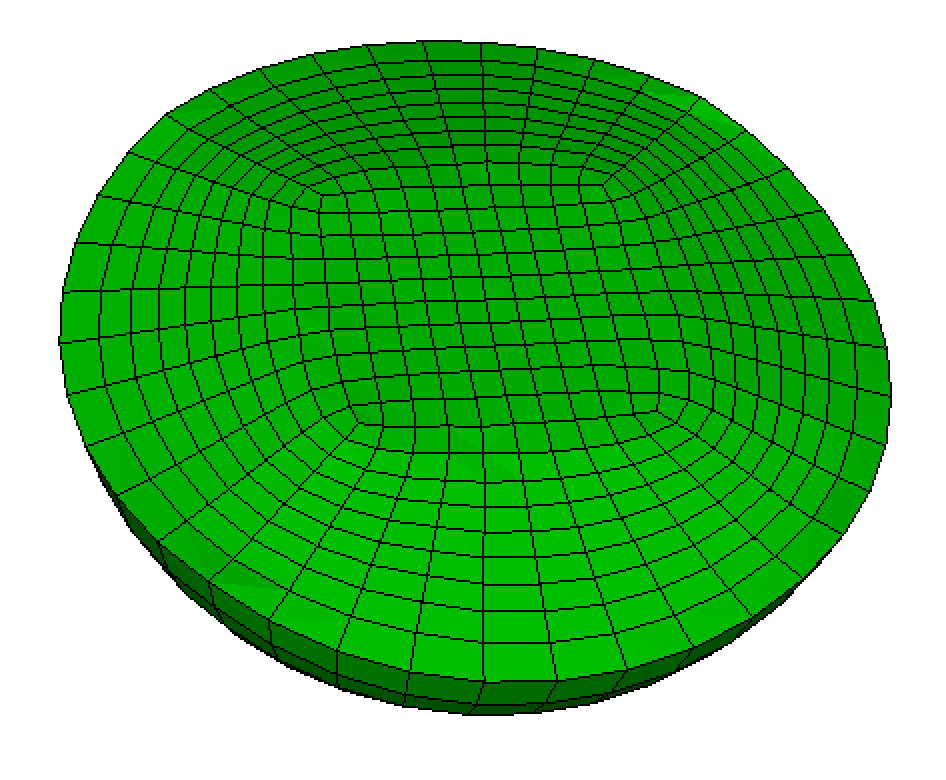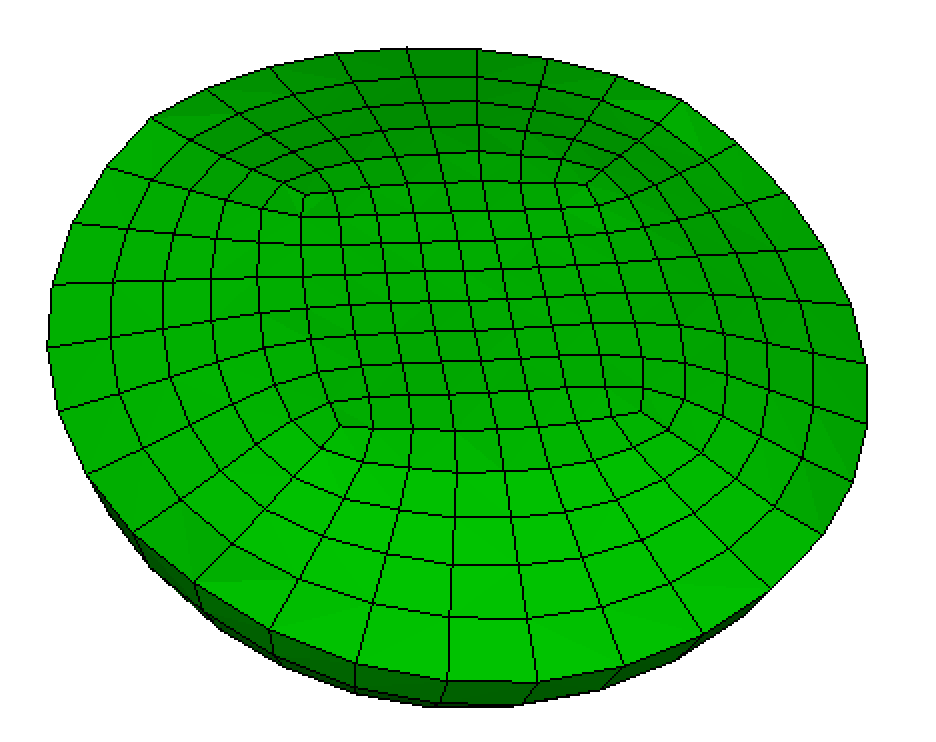# Sphere

Applies to: Volumes topologically equivalent to a sphere and having one surface.

Syntax:

Volume <range> Scheme Sphere [Graded_interval <int>] [Az_interval <int>] [Bias <val>] [Fraction <val>] [Max_smooth_iterations <int=2>]

Discussion:

This scheme generates a radially-graded mesh on a spherical volume having a single bounding surface. The mesh is a straightforward generalization of the circle scheme for surfaces. The mesh consists of an inner region and an outer region. The inner region is a mapped mesh of a cube and the outer region contains fronts that trasition from the cube surface to the sphere surface. The following describes the parameters that control the sphere mesh.

The number of intervals in the outer region from the inner mapped mesh to the surface of the sphere is controlled by the graded_interval input parameter. Azimuthal mesh lines in the outer portion of the sphere will have approximately constant radius. If graded_interval is not specified, a default number of intervals will be computed based on the interval size value assigned to the sphere volume.

Az_interval:

The number of azimuthal intervals around the equator is controlled by the az_interval input parameter. To maintain symmetry, the az_interval will be rounded to the nearest multiple of 8.

If az_interval is not specified, a default number of intervals will be computed either based on the the the interval value or on the mesh size value assigned to the volume. If the interval value is set (volume 1 interval 40, for example), the interval value will be used to define the number of azimuthal intervals. Otherwise, the mesh size will be used as the approximate size for elements on the inner mapped mesh.

Bias:

The bias parameter controls the amount of radial grading in the outer region of the mesh from the inner mapped mesh to the sphere surface. A bias = 1 will results in equal size intervals, while a bias < 1 will generate smaller intervals towards the sphere interior and a bias > 1 will generate smaller elements towards the sphere surface. If the bias parameter is not specified, a default bias will be computed so that element size gradually increases from the inner mapped mesh to the sphere surface. The default bias value will also be based on the interval size assigned to the sphere volume as it attempts to maintain approximately isotropic elements throughout the sphere.

Fraction:

The fraction parameter (between 0 and 1) determines what fraction of the sphere is occupied by the inner mapped mesh. The fraction is defined as ratio of the diagonal of the cube containing the mapped mesh to sphere's diameter. The default value for fraction is 0.5. Interval sizes in the inner mapped mesh are normally constrained by the az_intervals. If az_intervals are not specified, element sizes in this region will be based upon the interval size assigned to the sphere volume.

Max_smooth_iterations:

The Max_smooth_iterations parameter determines the number of smoothing iterations following initial definition of the sphere mesh. By default, the number of smoothing interations is set to 0, which will result in a symmetric mesh. Note that smoothing can improve the quality of the mesh, however, it may disturb the bias and fraction. When bias and fraction are critical then smoothing iterations should be set to 0.SPHERE MESH: fraction 0.3 graded_interval 6 az_interval 40 bias 0.8 max_smooth_iterations 0BIAS (uniform): fraction 0.3 graded_interval 6 az_interval 40 bias 1.0 max_smooth_iterations 0FRACTION: fraction 0.7 graded_interval 6 az_interval 40 bias 1.0 max_smooth_iterations 0INTERVAL: fraction 0.7 graded_interval 9 az_interval 40 bias 1.0 max_smooth_iterations 0SMOOTHING: fraction 0.7 graded_interval 9 az_interval 40 bias 1.0 max_smooth_iterations 2AZIMUTHAL (mesh coarseness): fraction 0.7 graded_interval 5 az_interval 32 bias 1.0 max_smooth_iterations 2BIAS (graded): fraction 0.9 graded_interval 9 az_interval 32 bias 1.5 max_smooth_iterations 0